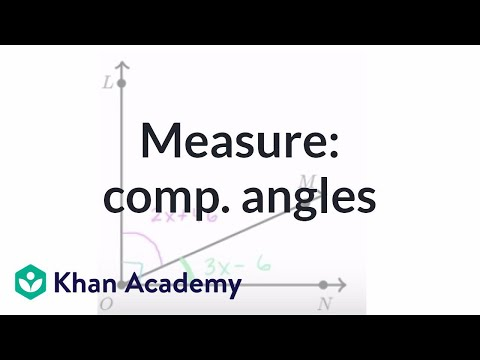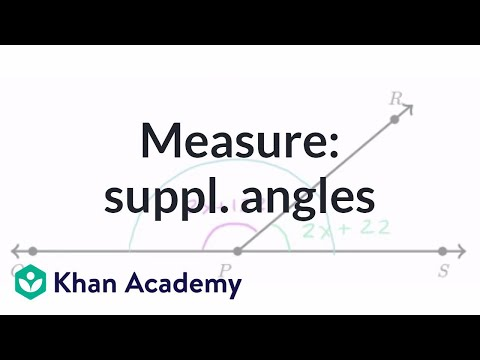Video

# Intro to two-step equations (Full video)

Description: Here's how we solve a two step equation. It begins with the concept of equality: what we do to one side of the equation must be done to the other. Created by Sal Khan. But before we even do that, I want you to think about a mathematical equation that can represent what is going on; that equates what we have on the left hand, with what we have on the right side of the scale. So let's think about what we have on the left side: we have 3 masses with mass X, so you can say we have 3x and then we have 2 masses of 1 kilogram, so in total we have 2 kg. So one way to think about the total mass on the left-hand side is 3x + 2.

### Other videos you might be interested in### Equation practice with complementary angles (Full video)